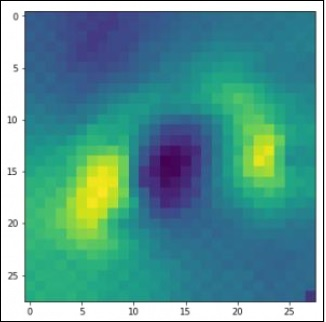# PyTorch - Visualization of Convents

In this chapter, we will be focusing on the data visualization model with the help of convents. Following steps are required to get a perfect picture of visualization with conventional neural network.

## Step 1

Import the necessary modules which is important for the visualization of conventional neural networks.

import os
import numpy as np
import pandas as pd
from sklearn.metrics import accuracy_score

import keras
from keras.models import Sequential, Model
from keras.layers import Dense, Dropout, Flatten, Activation, Input
from keras.layers import Conv2D, MaxPooling2D
import torch


## Step 2

To stop potential randomness with training and testing data, call the respective data set as given in the code below −

seed = 128
rng = np.random.RandomState(seed)
data_dir = "../../datasets/MNIST"
img_name = rng.choice(train.filename)
filepath = os.path.join(data_dir, 'train', img_name)


## Step 3

Plot the necessary images to get the training and testing data defined in perfect way using the below code −

pylab.imshow(img, cmap ='gray')
pylab.axis('off')
pylab.show()


The output is displayed as below −